06 285 000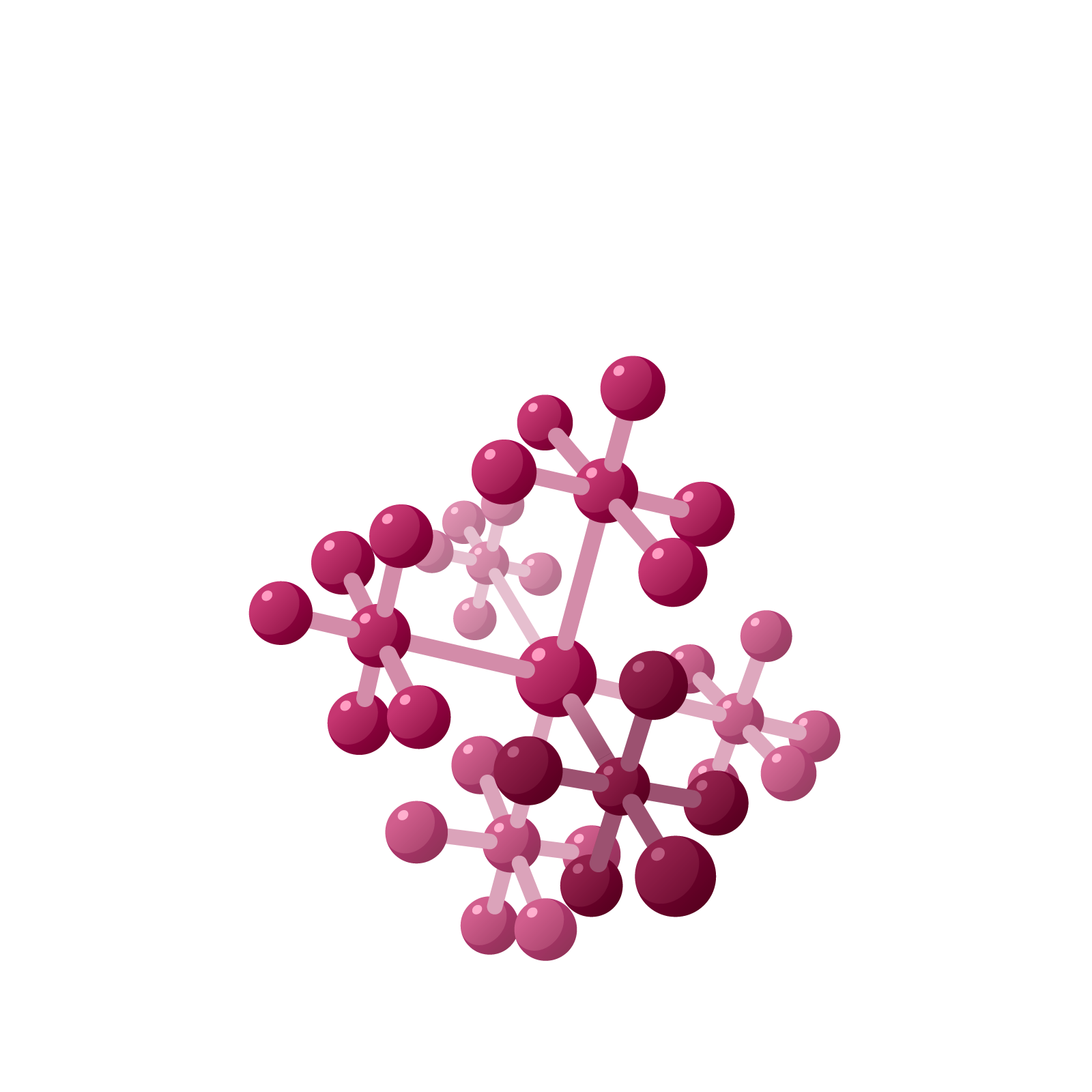# Station  11AGrape CodesThe six videos sprinkled throughout this lesson explain and demonstrate the text that immediately follow them.

Enjoy!## GRAPE CODES

Watch this Video: Grape Codes 1

Consider a row of dishes extending as far to the left as ever one desires, each labeled with a power of two, in order, starting from the right. In the picture I have six dishes.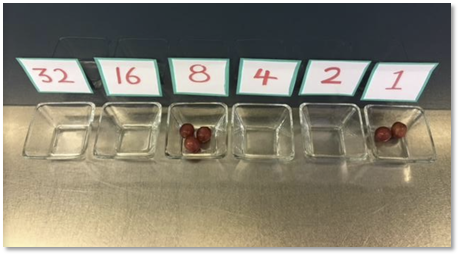Question 1: If I had ten dishes what would be the label of the leftmost dish?

I put grapes in my dishes and when I do each grape has value given by the label of the dish in which it sits. For example, three grapes in the $8$ dish and two in the $1$ dish together have a total value of $8+8+8+1+1=26$. I will write $3|0|0|2$ as a code for twenty-six. (I’ll ignore all leading zeros, that is, I won’t record the empty dishes to the left of the leftmost non-empty dish.) Other “grape codes” for twenty-six are possible.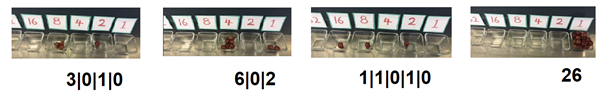Question 2: There happen to be a total of $114$ different grape codes for the number twenty-six. That is, there are $114$ different ways to represent the number twenty-six with grapes in dishes. The code $3|0|1|0$ represents the number twenty-six with just four grapes. The code $6|0|2$ uses eight grapes.

Of all 114 codes for twenty-six, is “$26$” (all twenty-six grapes in the $1$s bowl) the code that uses the most number of grapes? Is $1|1|0|1|0$ the code that uses the least number of grapes? How do you know?

Are there two different codes for twenty-six that use the same count of grapes? Are there five different codes that use the same count of grapes?

Watch this Video: Grape Codes 2

Question 3: Here are the first few numbers that have codes using only two grapes.

*$2, 3, 4, 5, 6, 8, 9, 10, 12, 16, 17, 18, 20, 24, 32, 33, 34, 36, \ldots$

What is the 50th number in this list?

Watch this Video: Grape Codes 3

Question 4: There are $6$ different grape codes for the number six.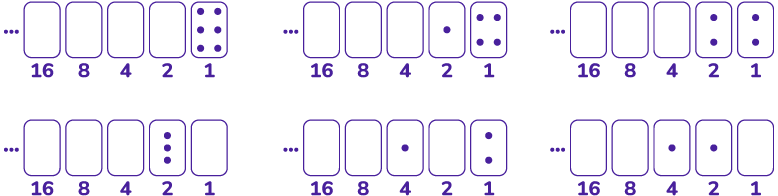a) Show that there are also $6$ grape codes for the number seven. Actually draw diagrams for each of the codes.

b) Is it true in general that the count of grape codes for an odd number is sure to equal the count of grape codes for the even number just before it?

## THINKING OF A $1 \leftarrow 2$ MACHINE

Watch this Video: Grape Codes 4

Question 5: A code for a number with at most one grape in each dish is called a binary code for that number. For instance, $1|1|0|1|0$ is a binary code for the number twenty-six and $1|1|0$ is a binary code for the number six.

a) Find a binary code for the number fifty.

b) Is every positive integer sure to have a binary code? (Read on!)

c) HARD CHALLENGE: Could a positive integer have two different binary codes?

In the story of Exploding Dots of the Global Math Project our row of dishes is simply a $1 \leftarrow 2$ machine.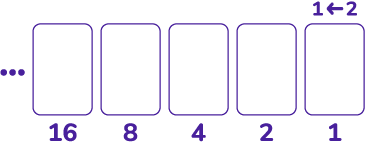>

One puts in dots (or grapes) in the rightmost box and let them “explode” in the following way:

Whenever there are two dots in a box, any box, they explode and disappear – KAPOW! – to be replaced by one dot, one box to the left.

And, indeed, two dots in any one box have the same combined value as one dot just to their left.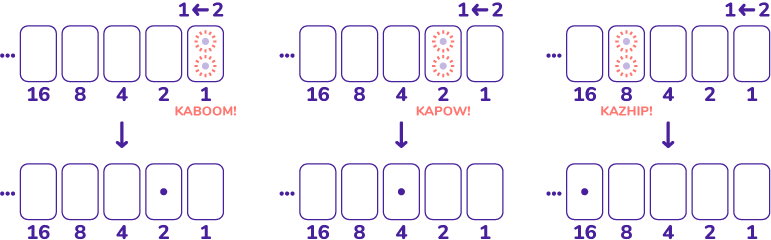In this way, placing a number of dots in the rightmost gives a representation of that number with at most one dot in each box. This proves that every positive integer has at least one binary code. For example, placing six dots into the machine eventually gives the binary code $1|1|0$ for the number $6$.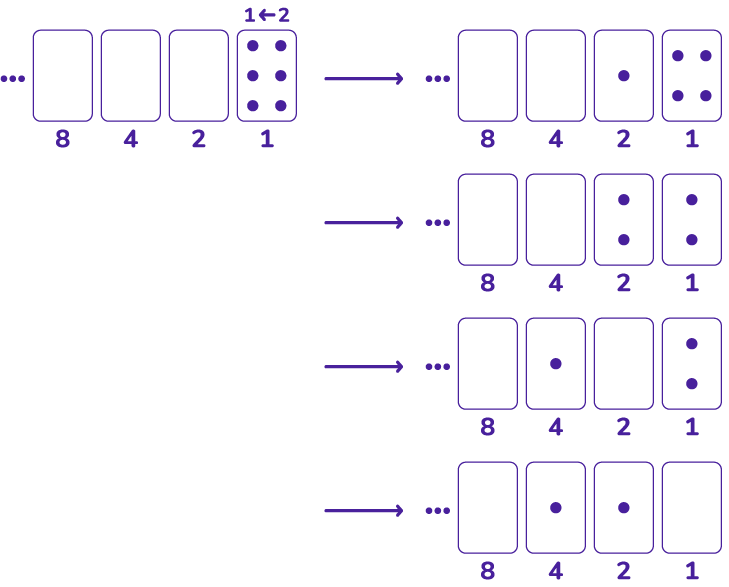Question 6: Which number has binary code $1|0|1|1$? Which number has binary code $1|0|1|1|0$ and which has code $1|0|1|1|1$?

Question 7:
a) Find the binary codes of the first twenty positive integers. What do you notice about the codes of the even numbers? The codes of the odd numbers?

b) Anouk says she invented a divisibility rule for the number $4$:

A number is divisible $4$ precisely when its binary code ends with two zeros.

Do you agree with her rule?

c) Is there a divisibility rule for the number based $3$ on the binary code of numbers?

Question 8:
a) Aba has a curious technique for finding the binary code of a number. She writes the number at the right of a page and halves it, writing the answer one place to its left, ignoring any fractions if the number was odd. She then repeats this process until she gets the number $1$. Then she writes $1$ under each odd number she sees and $0$ under each even number. The result is the binary code of the original number!

Here’s her work for computing the binary code of $22$.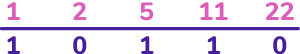Why does her technique work?

(HINT: Put $22$ dots in a $1 \leftarrow 2$ machine and watch what happens.)

b) FOLLOW-ON CHALLENGE:

Here’s a fun way to compute the product of two numbers, say, $22 \times 13$. Write the two numbers at the head of two columns, halve the left number (ignoring in fractions) and double the right number, and repeat until the number $1$ appears. Then cross out all the rows that have an even number on the left, and add all the numbers on the right that survive. That sum is the answer to the original product!

Why does this method work?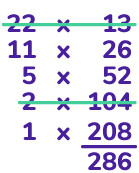(Hint: $22 = 16+4+2$ and $208+52+26=16 \times 13 + 4 \times 13 + 2 \times 13$.)

Question 9: Allistaire suggested that the binary code of $-1$ should be $\cdots 1|1|1|1|1$ (that is, an infinitely long string of ones going infinitely far to the left). He argued that adding one more dot to a $1 \leftarrow 2$ machine with a dot in each box produces, after explosions, an empty diagram: zero.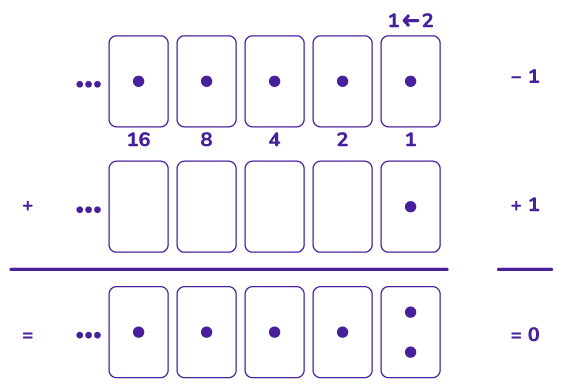Do you agree?

## PATHS THROUGH GRAPE CODES

Watch this Video: Grape Codes 5

The following diagram shows all the choices one can make when performing explosions on $6$ dots to lead to the binary code $1|1|0$ for the number $6$. The diagram also shows all ways we can represent $6$ with grapes!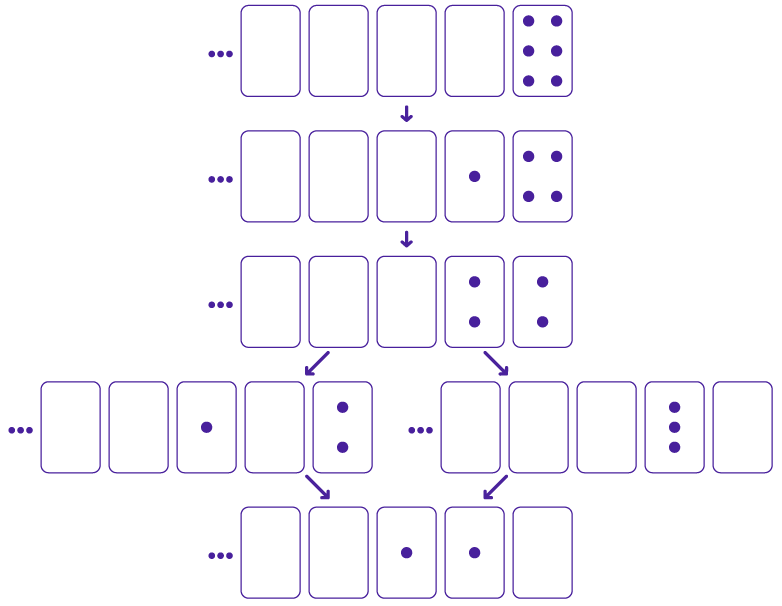Question 10:
a) Draw an analogous diagram for $12$ dots placed in a $1 \leftarrow 2$ machine. Show all the choices one can make for explosions and show that all paths lead to the same final binary code $1|1|0|0$.

b) There are $20$ ways to represent the number $12$ with grapes in dishes. Do all grape codes appear in your diagram? Do all paths of explosions lead to the same binary code of $1|1|0|0$?

c) In general, when one draws a diagram of all possible explosions for $N$ dots placed in a $1 \leftarrow 2$ machine, is the diagram sure to contain all the possible codes of $N$ with grapes? Do all paths lead to the same final binary code for $N$?

Question 11: Starting with $6$ dots in a $1 \leftarrow 2$ machine, one can perform a sequence of five explosions and “unexplosions” that produces all $6$ codes for $6$ in terms of grapes.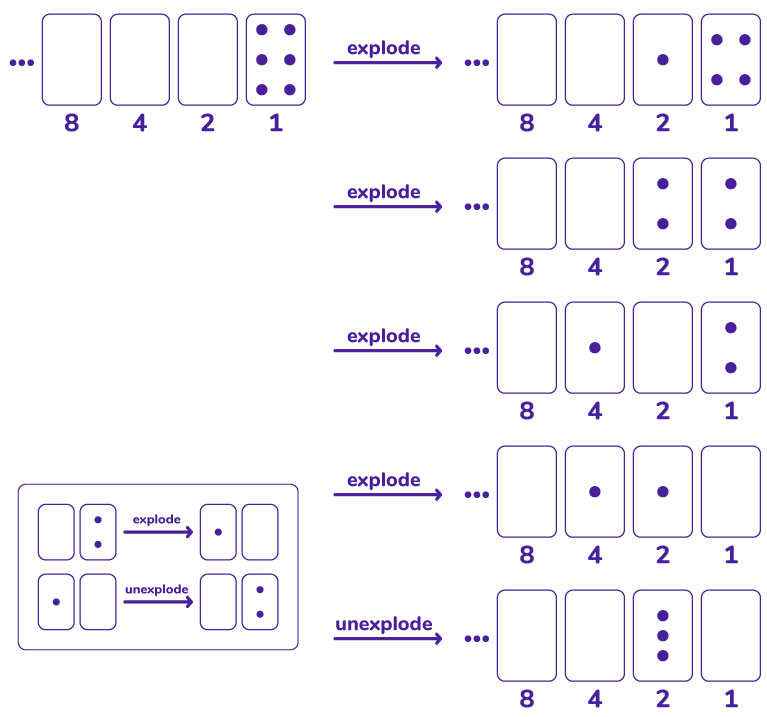It turns out that for any positive integer $N$ there is a sequence of explosions and unexplosions one can perform—starting with $N$ dots in the rightmost box of a $1 \leftarrow 2$ machine—to pass through all the possible grape codes f $N$ without repeating a code.

Starting with $12$ dots in the $1 \leftarrow 2$ machine, can you find a sequence of $19$ explosions and unexplosions that takes one through all $20$ possible codes for $12$ in terms of grapes?

## Counting Grape Codes

Watch this Video: Grape Codes 6

The table shows the number of different grape codes for the first few even numbers.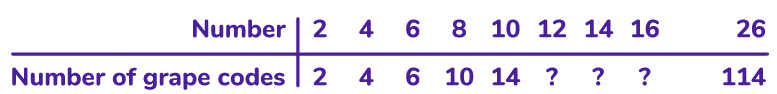Question 12:
a) Fill in the three missing entries. Care to find a few more entries?

b) Is there a pattern to the sequence of numbers you are finding? (And can you be sure any patterns you see are genuine?)

Comment: The 2018 ARML Power Question also explores these questions about codes for numbers, but not in the language of grapes nor Exploding Dots. To see full solutions to all the work here and its connection to the 2018 ARML Power Question, check out Visual Graphs of Binary Representations with Exploding Dots.

### Let's Go Wild!